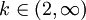# Example: Integral Test with a Logarithm

Previous: Integral Test Example

Next: The p-series

## Problem

Using the integral test, determine whether the infinite seriesconverges or diverges, or if the test cannot be applied.

## Complete Solution

First we must establish whether or not the test can be applied. If we letthen we much check if f(x) is continuous, decreasing, and positive.

### Step (1): Check Continuity

Both the numerator and denominator are continuous functions on (2,∞), so their ratio will also be continuous. So f is continuous.

### Step (2): Check Positivity

Both the numerator and denominator are positive functions on (2,∞), so their ratio will also be positive. So f is positive.

### Step (3): Check to See if f is Decreasing

We can apply the usual first derivative test:The above quantity is negative forso we have that our function is also decreasing on this interval.

### Step (4): Apply Integral Test

Since f is positive, continuous, and decreasing, we can apply the integral test.Therefore, the infinite series diverges, because the above integral diverges.

## Discussion of Each Step

### Step (1) and (2)

These checks must be done, but are, in this example, straightforward.

### Step (3)

Recall that the first derivative test tells us that a function is decreasing on an interval if the first derivative of that function is negative everywhere on that interval. Using the quotient rule to calculate our derivative, we find that indeed, the function is decreasing on (2,∞).

## Potential Challenges

### Getting Started

Given that the question asks us to apply the integral test, we should know immediately how to get started: check for continuity, positivity, and if our function is decreasing. These three checks must always be performed before the test can be applied.

Previous: Integral Test Example

Next: The p-series